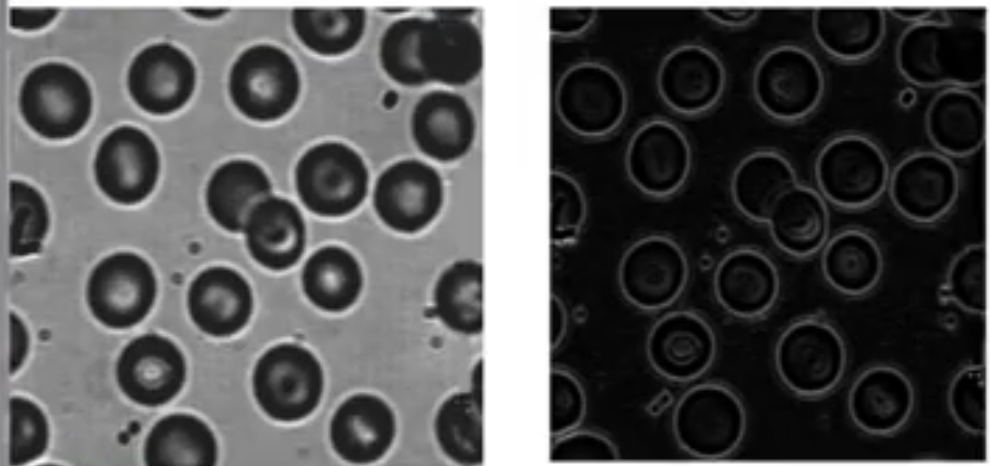2015-02-06 10:02:21 xiaojun111111 阅读数 1016

int Height = bmp_old.Height;
int Width = bmp_old.Width;
Bitmap bitmap = new Bitmap(Width, Height);
Bitmap MyBitmap = (Bitmap)bmp_old;
Color pixel;
//拉普拉斯模板
int[] Laplacian ={ -1, -1, -1, -1, 9, -1, -1, -1, -1 };
for (int x = 1; x < Width - 1; x++)
for (int y = 1; y < Height - 1; y++)
{
int r = 0, g = 0, b = 0;
int Index = 0;

for (int col = -1; col <= 1; col++)
for (int row = -1; row <= 1; row++)
{
pixel = MyBitmap.GetPixel(x + row, y + col);
r += pixel.R * Laplacian[Index];
g += pixel.G * Laplacian[Index];
b += pixel.B * Laplacian[Index];
Index++;
}
//处理颜色值溢出
r = r > 255 ? 255 : r;
r = r < 0 ? 0 : r;
g = g > 255 ? 255 : g;
g = g < 0 ? 0 : g;
b = b > 255 ? 255 : b;
b = b < 0 ? 0 : b;
bitmap.SetPixel(x - 1, y - 1, Color.FromArgb(r, g, b));
}

Form3 output2 = new Form3(bitmap, "锐化");
output2.MdiParent = this;
output2.Show();

2019-12-23 13:55:40 weixin_44244154 阅读数 54

# 实验内容

## 1.1 单方向梯度算子锐化：

### 水平方向锐化：clc;
%I1=rgb2gray(I); %将彩色图变成灰色图
subplot(1,3,1);
imshow(I1),title('原图');

mode=[1 2 1;0 0 0;-1 -2 -1];%水平方向锐化模板
[m,n]=size(I1);
I2=double(I1);
for i=2:m-1
for j=2:n-1
I2(i,j)=2*I1(i,j)+I1(i-1,j-1)+I1(i-1,j+1)-I1(i+1,j+1)-2*I1(i+1,j)-I1(i+1,j);
end
end
subplot(1,3,2);
imshow(I2),title('边缘提取后的图像');

I3=I1;
for i=2:m-1
for j=2:n-1
I3(i,j)=2*I1(i,j)+I1(i-1,j-1)+I1(i-1,j+1)-I1(i+1,j+1)-2*I1(i+1,j)-I1(i+1,j)+I1(i,j);
end
end
subplot(1,3,3);
imshow(uint8(I3)),title('锐化后的图像');



### 竖直方向锐化：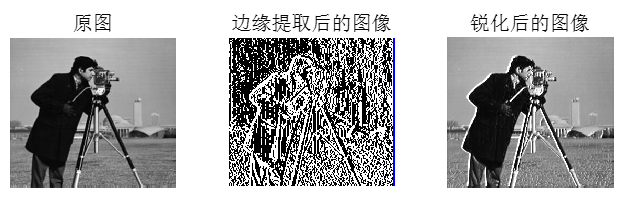clc;
%I1=rgb2gray(I); %将彩色图变成灰色图
subplot(1,3,1);
imshow(I1),title('原图');
mode=[1 0 -1;2 0 -2;1 0 -1];%竖直方向锐化模板
[m,n]=size(I1);
I2=double(I1);
for i=2:m-1
for j=2:n-1
I2(i,j)=I1(i-1,j-1)-I1(i-1,j+1)+2*I1(i,j-1)-2*I1(i,j+1)+I1(i+1,j-1)-I1(i+1,j+1);
end
end
subplot(1,3,2);
imshow(I2),title('边缘提取后的图像');

I3=I1;
for i=2:m-1
for j=2:n-1
I3(i,j)=I1(i-1,j-1)-I1(i-1,j+1)+2*I1(i,j-1)-2*I1(i,j+1)+I1(i+1,j-1)-I1(i+1,j+1)+I1(i,j);
end
end
subplot(1,3,3);
imshow(uint8(I3)),title('锐化后的图像');



## 1.2 Robert算子锐化：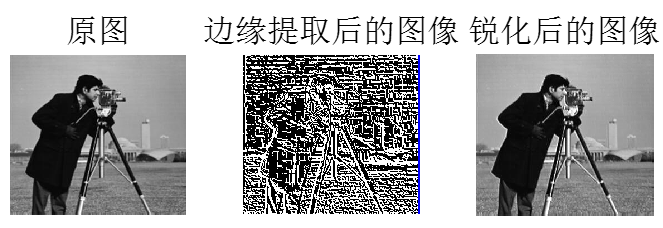Robert算子对细节反映较敏感，但提取到的信息量较少，中心位置不明显，图像处理后的边缘不是很平滑。Roberts算子采用对角线方向相邻两像素之差近似梯度幅值检测边缘。检测水平和垂直边缘的效果好于斜向边缘，定位精度高，对噪声敏感。

clc;
%I1=rgb2gray(I); %将彩色图变成灰色图
subplot(1,3,1);
imshow(I1),title('原图','fontsize',16);

mode=[0,-1;
1,0];
[m,n]=size(I1);
I2=double(I1);
for i=2:m-1
for j=2:n-1
I2(i,j)=I1(i+1,j)-I1(i,j+1);
end
end

subplot(1,3,2);
imshow(I2),title('边缘提取后的图像','fontsize',16);
I3=I1;
for i=2:m-1
for j=2:n-1
I3(i,j)=I1(i+1,j)-I1(i,j+1)+I1(i,j);
end
end
subplot(1,3,3);
imshow(uint8(I3)),title('锐化后的图像','fontsize',16);



## 1.3 Sobel算子锐化：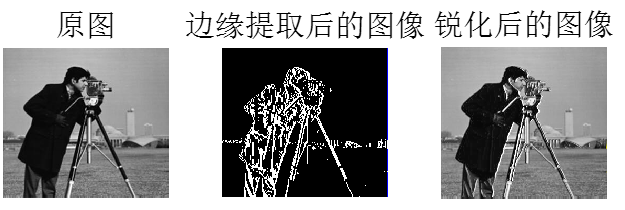Sobel算子主要用作边缘检测，在技术上，它是一离散性差分算子，用来运算图像亮度函数的灰度之近似值。在图像的任何一点使用此算子，将会产生对应的灰度矢量或是其法矢量。Sobel算子根据像素点上下、左右邻点灰度加权差，在边缘处达到极值这一现象检测边缘。对噪声具有平滑作用，提供较为精确的边缘方向信息，边缘定位精度不够高。当对精度要求不是很高时，是一种较为常用的边缘检测方法。Sobel算子引入了平均因素，增强了最近的像素影响，噪声抑制效果比Prewitt算子要好。

clc;
%I1=rgb2gray(I); %将彩色图变成灰色图
subplot(1,3,1);
imshow(I1),title('原图','fontsize',16);
mode=[-1,0,1;
-2,0,2;
-1,0,1];

[m,n]=size(I1);
I2=double(I1);
for i=2:m-1
for j=2:n-1
I2(i,j)=I1(i+1,j+1)+2*I1(i,j+1)-I1(i+1,j-1)+I1(i-1,j+1)-2*I1(i,j-1)-I1(i-1,j-1);
end
end
subplot(1,3,2);
imshow(I2),title('边缘提取后的图像','fontsize',16);
I3=double(I1);
for i=2:m-1
for j=2:n-1
I3(i,j)=I1(i+1,j+1)+2*I1(i,j+1)-I1(i+1,j-1)+I1(i-1,j+1)-2*I1(i,j-1)-I1(i-1,j-1)+I1(i,j);
end
end
subplot(1,3,3);
imshow(uint8(I3)),title('锐化后的图像','fontsize',16);



## 1.4 Priwiff算子锐化：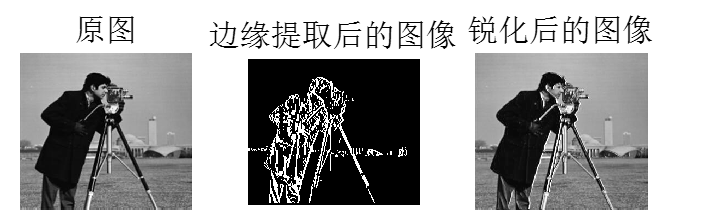Prewitt算子利用像素点上下、左右邻点灰度差，在边缘处达到极值检测边缘。对噪声具有平滑作用，定位精度不够高。Priwiff算子对噪声相对不敏感，有抑制噪声作用，但操作简便，计算方便快捷。

clc
I1=rgb2gray(I); %将彩色图变成灰色图
subplot(1,3,1);
imshow(I1),title('原图','fontsize',16);

model=[-1,0,1;
-1,0,1;
-1,0,1];
[m,n]=size(I1);
I2=zeros(288,340);

for i=2:m-1
for j=2:n-1
I2(i,j)=I1(i-1,j+1)+I1(i,j+1)+I1(i+1,j+1)-I1(i-1,j-1)-I1(i,j-1)-I1(i+1,j-1);
end
end

subplot(1,3,2);
imshow(I2),title('边缘提取后的图像','fontsize',16);
I3=I1;
for i=2:m-1
for j=2:n-1
I3(i,j)=I1(i-1,j+1)+I1(i,j+1)+I1(i+1,j+1)-I1(i-1,j-1)-I1(i,j-1)-I1(i+1,j-1)+I1(i,j);
end
end
subplot(1,3,3);
imshow(uint8(I3)),title('锐化后的图像','fontsize',16);



## 1.5 Laplacian算子锐化：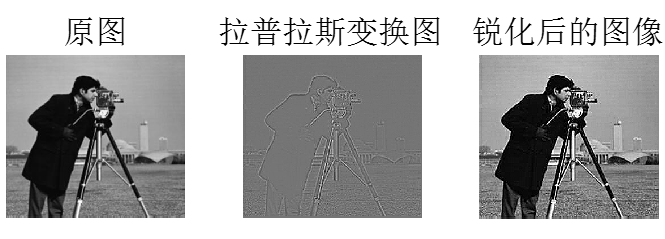Laplace算子是一种各向同性算子，二阶微分算子，在只关心边缘的位置而不考虑其周围的象素灰度差值时比较合适。Laplace算子对孤立象素的响应要比对边缘或线的响应要更强烈，因此只适用于无噪声图象。存在噪声情况下，使用Laplacian算子检测边缘之前需要先进行低通滤波。所以，通常的分割算法都是把Laplacian算子和平滑算子结合起来生成一个新的模板。

clc;clear;
f = double(f);
[row,col] = size(f);

%%
L = [0 -1 0;-1 4 -1;0 -1 0];%可以使用模板，用模板内像素值组成矩阵点乘算子；也可直接使用公式形式。
g = zeros(row,col);
for i=2:row-1
for j=2:col-1%求和值必须新图，否则影响后面求和结果
g(i,j) = sum(sum([f(i-1,j-1) f(i-1,j) f(i-1,j+1); ...
f(i,j-1) f(i,j) f(i,j+1); ...
f(i+1,j-1) f(i+1,j) f(i+1,j+1)].*L));
end
end
g1 = zeros(row,col);
for i=1:row
for j=1:col%求和值必须新图，否则影响后面求和结果
g1(i,j) = g(i,j)+f(i,j);

end
end

%%
g=mat2gray(g);
subplot(1,3,1),imshow(uint8(f)),title('原图');
subplot(1,3,2),imshow(g),title('拉普拉斯变换图');
subplot(1,3,3),imshow(uint8(g1)),title('锐化后的图像');


## 1.6 LOG算子锐化：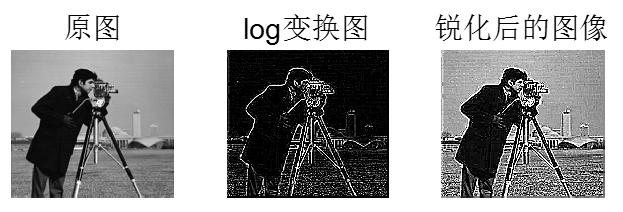**LOG算子对边缘噪声十分敏感。该方法是将高斯滤波和拉普拉斯检测算子结合在一起进行边缘检测的方法，此种方法在边缘检测前首先通过滤波消除噪声，因此检测效果非常好，图像处理后也很清晰。LOG滤波器方法通过检测二阶导数过零点来判断边缘点。LOG滤波器中的a正比于低通滤波器的宽度，a越大，平滑作用越显著，去除噪声越好，但图像的细节也损失越大，边缘精度也就越低。所以在边缘定位精度和消除噪声级间存在着矛盾，应该根据具体问题对噪声水平和边缘点定位精度要求适当选取。
Robert算子定位比较精确，但由于不包括平滑，所以对于噪声比较敏感。Prewitt算子和Sobel算子都是一阶的微分算子，而前者是平均滤波，后者是加权平均滤波且检测的图像边缘可能大于2个像素。这两者对灰度渐变低噪声的图像有较好的检测效果，但是对于混合多复杂噪声的图像，处理效果就不理想了。 **

clc;
clear;
f=rgb2gray(I); %将彩色图变成灰色图
mode=[0 0 -1 0 0;
0 -1 -2 -1 0;
-1 -2 16 -2 -1;
0 -1 -2 -1 0;
0 0 -1 0 0];%构造LOG算子

[m,n]=size(f);
f=double(f);
g = zeros(m,n);
for i=3:m-2
for j=3:n-2
g(i,j) = sum(sum([f(i-2,j-2) f(i-2,j-1) f(i-2,j) f(i-2,j+1) f(i-2,j+2); ...
f(i-1,j-2) f(i-1,j-1) f(i-1,j) f(i-1,j+1) f(i-1,j+2); ...
f(i,j-2) f(i,j-1) f(i,j) f(i,j+1) f(i,j+2);...
f(i+1,j-2) f(i+1,j-1) f(i+1,j) f(i+1,j+1) f(i+1,j+2);...
f(i+2,j-2) f(i+2,j-1) f(i+2,j) f(i+2,j+1) f(i+2,j+2)].*mode));
end
end
g1 = zeros(m,n);
for i=1:m
for j=1:n%求和值必须新图，否则影响后面求和结果
g1(i,j) = g(i,j)+f(i,j);

end
end
%%
%g=mat2gray(g);
subplot(1,3,1),imshow(uint8(f)),title('原图','fontsize',16);
subplot(1,3,2),imshow(uint8(g)),title('log变换图','fontsize',16);
subplot(1,3,3),imshow(uint8(g1)),title('锐化后的图像','fontsize',16);


2019-09-20 00:02:19 weixin_44225182 阅读数 2361

## 图像锐化

Sobel算子

 I=imread('a2.jpg'); %读取图像
I1=rgb2gray(I); %将彩色图变成灰色图
imshow(I1),title('原图');

model=[-1,0,1;
-2,0,2;
-1,0,1];
[m,n]=size(I1);
I2=double(I1);

for i=2:m-1
for j=2:n-1
I2(i,j)=I1(i+1,j+1)+2*I1(i+1,j)+I1(i+1,j-1)-I1(i-1,j+1)-2*I1(i-1,j)-I1(i-1,j-1);
end
end
figure,
imshow(I2),title('边缘提取后的图像');
I2 = I2 + double(I1);
figure,
imshow(uint8(I2)),title('锐化后的图像');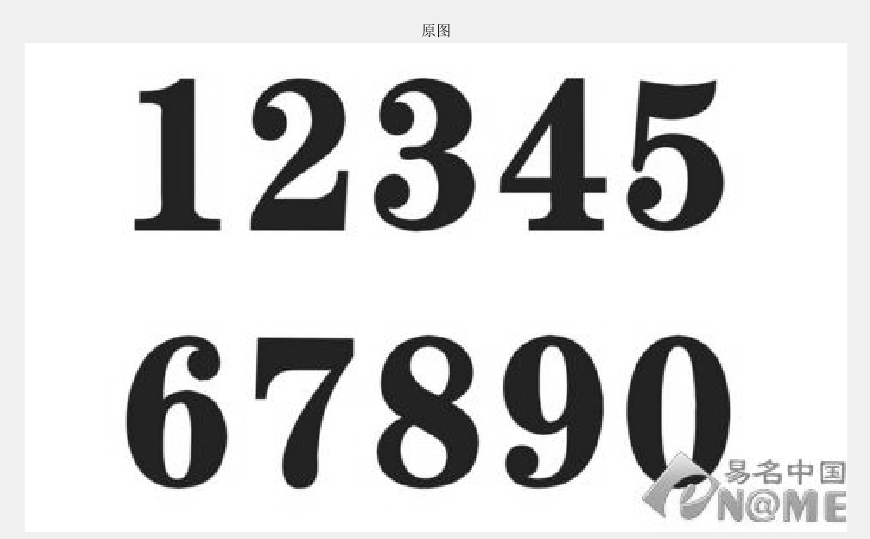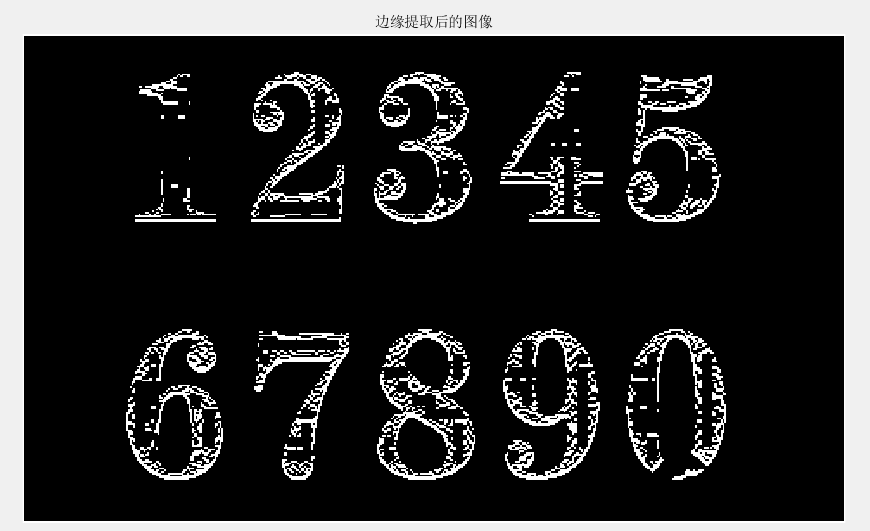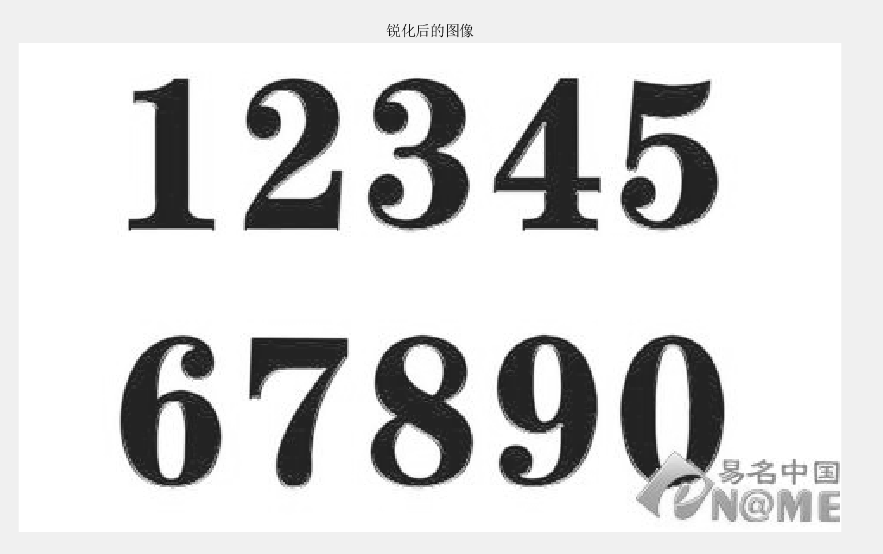Robert算子

 I=imread('a2.jpg'); %读取图像
I1=rgb2gray(I); %将彩色图变成灰色图
imshow(I1),title('原图');
model=[0,-1;1,0];
[m,n]=size(I1);
I2=double(I1);
for i=2:m-1
for j=2:n-1
I2(i,j)=I1(i+1,j)-I1(i,j+1);
end
end
figure,imshow(I2),title('边缘提取后的图像');
I2 = I2 + double(I1);

figure,
imshow(uint8(I2)),title('锐化后的图像');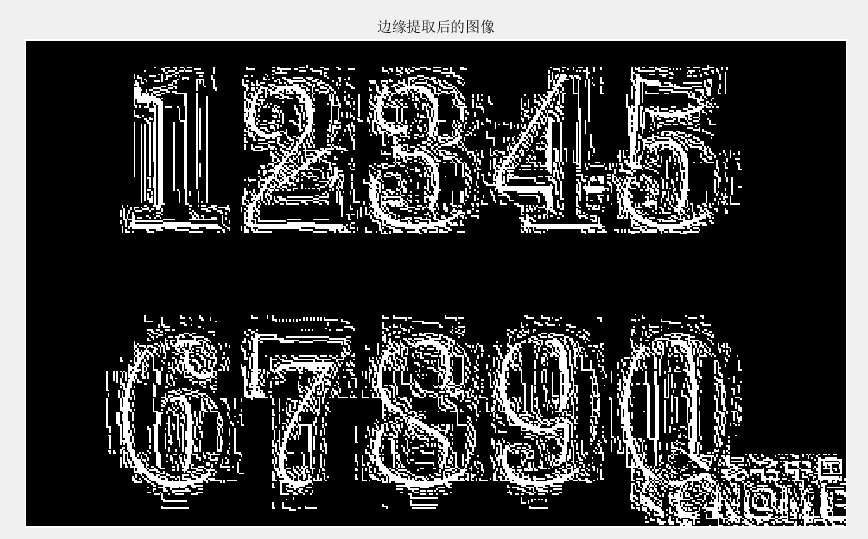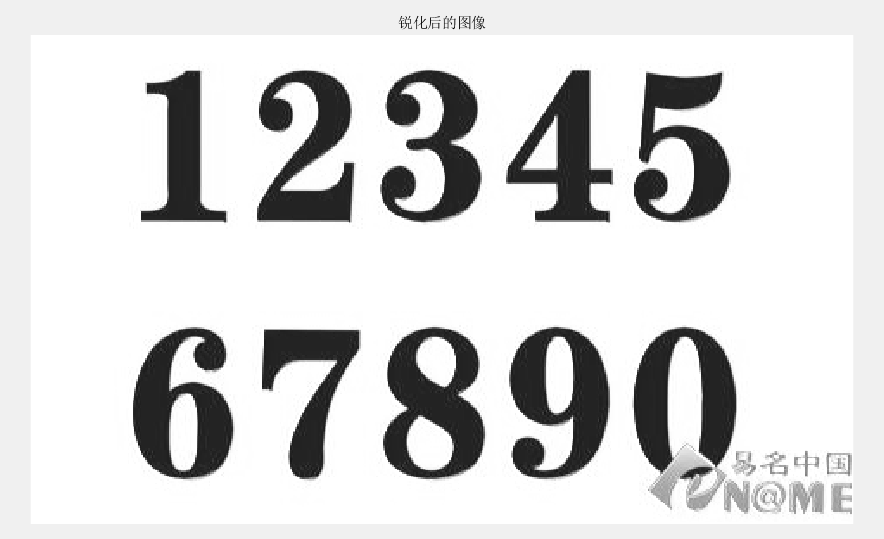Prewitt算子

 I=imread('a2.jpg');
I1=rgb2gray(I); %将彩色图变成灰色图
subplot(1,2,1);
imshow(I1),title('原图');

model=[-1,0,1;
-1,0,1;
-1,0,1];
[m,n]=size(I1);

I2=I1;
for i=2:m-1
for j=2:n-1
tem=I1(i-1:i+1,j-1:j+1);
tem=double(tem).*double(model);

I2(i,j)=sum(sum(tem));

end
end

figure,
imshow(uint8(I2)),title('边缘提取后的图像');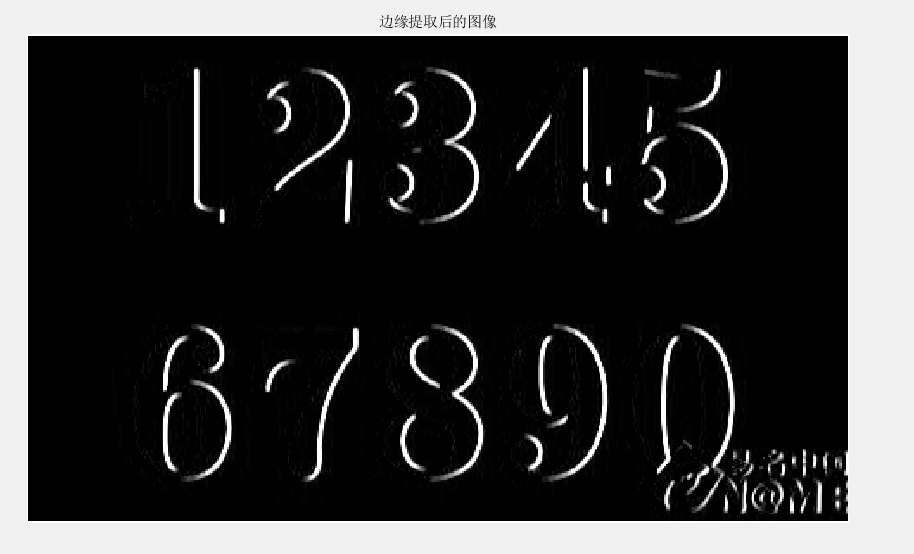laplacian算子

 I=imread('C:\Users\LiCongliang\Desktop\数字图像处理\数字图像处理第五次作业\tea.png');
I1=mat2gray(I);%实现图像矩阵的归一化操作
[m,n]=size(I1);
newGrayPic=I1;%为保留图像的边缘一个像素
LaplacianNum=0;%经Laplacian算子计算得到的每个像素的值
LaplacianThreshold=0.2;%设定阈值
for j=2:m-1 %进行边界提取
for k=2:n-1
LaplacianNum=abs(4*I1(j,k)-I1(j-1,k)-I1(j+1,k)-I1(j,k+1)-I1(j,k-1));
if(LaplacianNum > LaplacianThreshold)
newGrayPic(j,k)=255;
else
newGrayPic(j,k)=0;
end
end
end
I2=rgb2gray(I); %将彩色图变成灰色图
imshow(I2),title('原图');
figure,
imshow(newGrayPic);
title('Laplacian算子的处理结果')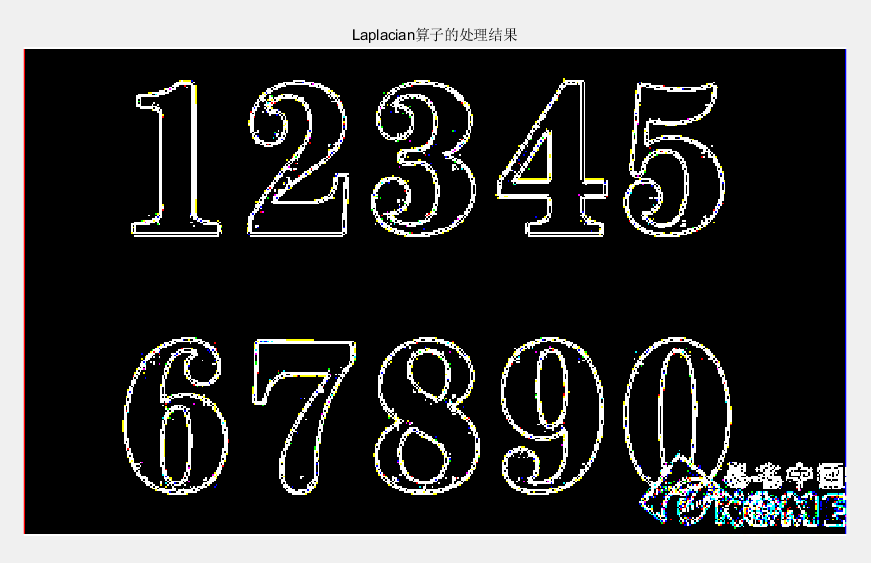## 更多

2019-12-12 22:40:32 weixin_44225182 阅读数 241

## 二、实验目的

1.熟悉MATLAB软件的使用。
2.掌握图像锐化的原理及数学运算。
3.于MATLAB环境下编程实现对图片锐化。

## 三、实验内容

利用图像基于单方向梯度算子，Robert算子，Sobel算 子，Pritt算 子, Laplacian算子，多方向模板，LoG算子编写matlab增强程序;最后，显示边缘图像和锐化增强图像，并对这些仿真结果所呈现的边缘提取和增强效果进行对比分析。

Win10 64位电脑
MATLAB2017a

## 五、实验原理

1.Sobel算子是像素图像边缘检测中最重要的算子之一。在技术上，它是一个离散的一阶差分算子，用来计算图像亮度函数的一阶梯度之近似值。在图像的任何一点使用此算子，将会产生该点对应的梯度矢量或是其法矢量。
2.Roberts边缘检测算子是一种利用局部差分算子寻找边缘的算子，Robert算子图像处理后结果边缘不是很平滑。经分析，由于Robert算子通常会在图像边缘附近的区域内 产生较宽的响应，故采用上述算子检测的边缘图像常需做细化处理，边缘定位的精度不是很高。
3.Prewitt算子是一种一阶微分算子的边缘检测，利用像素点上下、左右邻点的灰度差，在边缘处达到极值检测边缘，去掉部分伪边缘，对噪声具有平滑作用 。其原理是在图像空间利用两个方向模板与图像进行邻域卷积来完成的，这两个方向模板一个检测水平边缘，一个检测垂直边缘。
(1) f的拉普拉斯算子也是笛卡尔坐标系xi中的所有非混合二阶偏导数求和：
(2) 作为一个二阶 微分算子，拉普拉斯算子把C函数映射到C函数，对于k ≥ 2。

## 六、实验过程及代码

Sobel算子

 I=imread('a3.jpg'); %读取图像
I1=rgb2gray(I); %将彩色图变成灰色图
subplot(131),imshow(I1),title('原图');

model=[-1,0,1;
-2,0,2;
-1,0,1];
[m,n]=size(I1);
I2=double(I1);

for i=2:m-1
for j=2:n-1
I2(i,j)=I1(i+1,j+1)+2*I1(i+1,j)+I1(i+1,j-1)-I1(i-1,j+1)-2*I1(i-1,j)-I1(i-1,j-1);
end
end
subplot(132),
imshow(I2),title('边缘提取后的图像');
I2 = I2 + double(I1);
subplot(133),
imshow(uint8(I2)),title('锐化后的图像');


Robert算子

 I=imread('a3.jpg'); %读取图像
I1=rgb2gray(I); %将彩色图变成灰色图
subplot(131),imshow(I1),title('原图');
model=[0,-1;1,0];
[m,n]=size(I1);
I2=double(I1);
for i=2:m-1
for j=2:n-1
I2(i,j)=I1(i+1,j)-I1(i,j+1);
end
end
subplot(132),imshow(I2),title('边缘提取后的图像');
I2 = I2 + double(I1);

subplot(133),
imshow(uint8(I2)),title('锐化后的图像');


Prewitt算子

 I=imread('a3.jpg');
I1=rgb2gray(I); %将彩色图变成灰色图
subplot(131);
imshow(I1),title('原图');
model=[-1,0,1;
-1,0,1;
-1,0,1];
[m,n]=size(I1);
I2=I1;
for i=2:m-1
for j=2:n-1
tem=I1(i-1:i+1,j-1:j+1);
tem=double(tem).*model;

I2(i,j)=sum(sum(tem));
end
end
subplot(132),
imshow(uint8(I2)),title('边缘提取后的图像');
I2=I2+I1;
subplot(133),
imshow(I2),title('图像锐化')


laplacian算子

I=imread('a3.jpg');
I1=mat2gray(I);%实现图像矩阵的归一化操作
[m,n]=size(I1);
newGrayPic=I1;%为保留图像的边缘一个像素
LaplacianNum=0;%经Laplacian算子计算得到的每个像素的值
LaplacianThreshold=0.2;%设定阈值
for j=2:m-1 %进行边界提取
for k=2:n-1
LaplacianNum=abs(4*I1(j,k)-I1(j-1,k)-I1(j+1,k)-I1(j,k+1)-I1(j,k-1));
if(LaplacianNum > LaplacianThreshold)
newGrayPic(j,k)=255;
else
newGrayPic(j,k)=0;
end
end
end
I2=rgb2gray(I); %将彩色图变成灰色图
subplot(131),imshow(I2),title('原图');
subplot(132),
imshow(newGrayPic);
title('Laplacian算子的处理结果')
t=I1+newGrayPic;
subplot(133),imshow(t),title('图像锐化后')


Log算子

 t=imread('a3.jpg');
t=rgb2gray(t);
[m,n]=size(t);
subplot(1,3,1),imshow(t),title('原图')
tt=t;
model=[0,0,1,0,0;
0,1,2,1,0;
1,2,-16,2,1;
0,0,1,0,0;
0,1,2,1,0;]

for i=3:m-2
for j=3:n-2
tem=double(t(i-2:i+2,j-2:j+2)).*model;
x=sum(sum(tem));
tt(i,j)=x;
end
end

subplot(1,3,2),imshow(tt),title('log提取边缘后')
t_1=double(tt)+double(t);
subplot(1,3,3),imshow(uint8(t_1)),title('log锐化后')


## 七、实验结果与分析

Sobel算子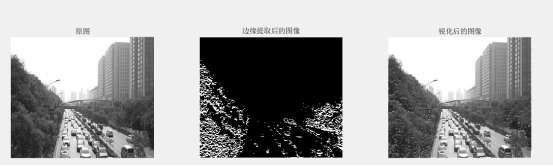Robert算子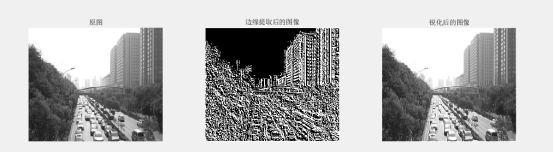Prewitt算子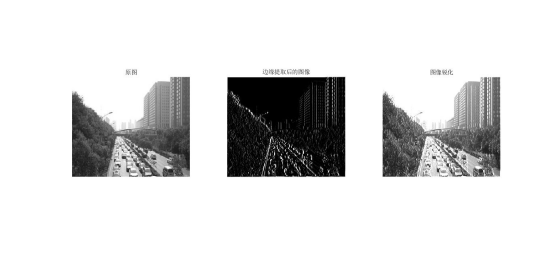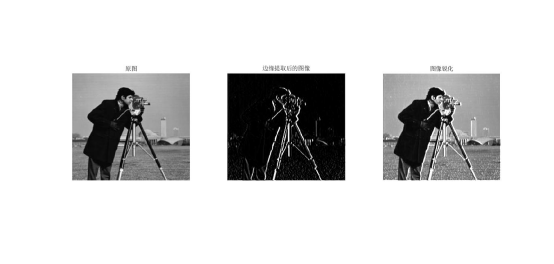laplacian算子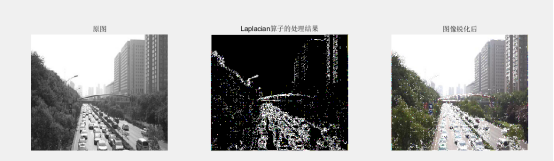Log算子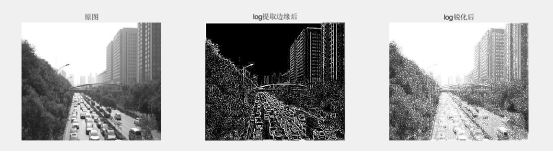图像锐化(image sharpening)是补偿图像的轮廓，增强图像的边缘及灰度跳变的部分，使图像变得清晰，分为空间域处理和频域处理两类。图像锐化是为了突出图像上地物的边缘、轮廓，或某些线性目标要素的特征。这种滤波方法提高了地物边缘与周围像元之间的反差，因此也被称为边缘增强。我的理解：图像锐化就是通过使得图像的边缘更加突出，可以是原图与原图的高频部分叠加而成。
Sobel算法与Priwitt算法的思路相同，属于同一类型，因此处理效果基本相同。Roberts算法的模板为2*2，提取信息较弱。单方向锐化经过处理之后，也可以对边界进行增强。Laplacian算子对噪声比较敏感，Laplacian算子有一个缺点是它对图像中的某些边缘产生双重响应。所以图像一般先经过平滑处理，通常把Laplacian算子和平滑算子结合起来生成一个新的模板-Log算子。

## 八、实验总结及心得体会

通过此次实验，自己学习了图像锐化的几种算子。开始的时候，不懂每个算子的来源，后面看了老师的推导过程后，发现其实锐化就是先对图像进行差分（类似求导），再叠加到原图上。这里主要的问题就是对算子的差分原理的验证，然后在MATLAB上机过程中，没有注意到数据类型的限制，导致数据上溢。

## 更多

2019-02-13 11:32:14 dzm123lalala 阅读数 618

# 图像边缘锐化基本方法

• 微分运算
• 梯度锐化
• 边缘检测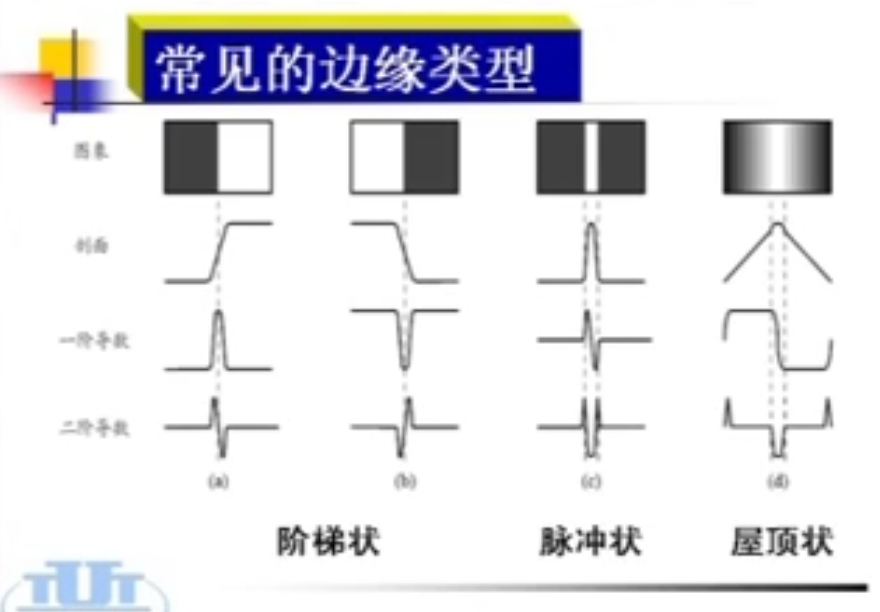## 用差分定义一元函数f(x)的一阶微分：

$\frac{\partial f}{\partial x} =f(x+1)-f(x)$

## 用差分定义一元函数f(x)的二阶微分：

$\frac{\partial^2 f}{\partial x^2} =f(x+1)+f(x-1)-2f(x)$

## 梯度运算

$\bigtriangledown t=\begin{bmatrix} G_x \\ G_y \\ \end{bmatrix}=\begin{bmatrix} \frac{\partial f}{\partial x} \\ \frac{\partial f}{\partial y} \\ \end{bmatrix}$
$\bigtriangledown f \approx |G_x|+|G_y|$

$\bigtriangledown f=\sqrt{(\frac{\partial f}{\partial x})^2+(\frac{\partial f}{\partial y})^2}$

$|\bigtriangledown f_(\infty_) |=max \lbrace |\frac{\partial f}{\partial x}|,|\frac{\partial f}{\partial y}| \rbrace$

## Robert提出的交叉微分算子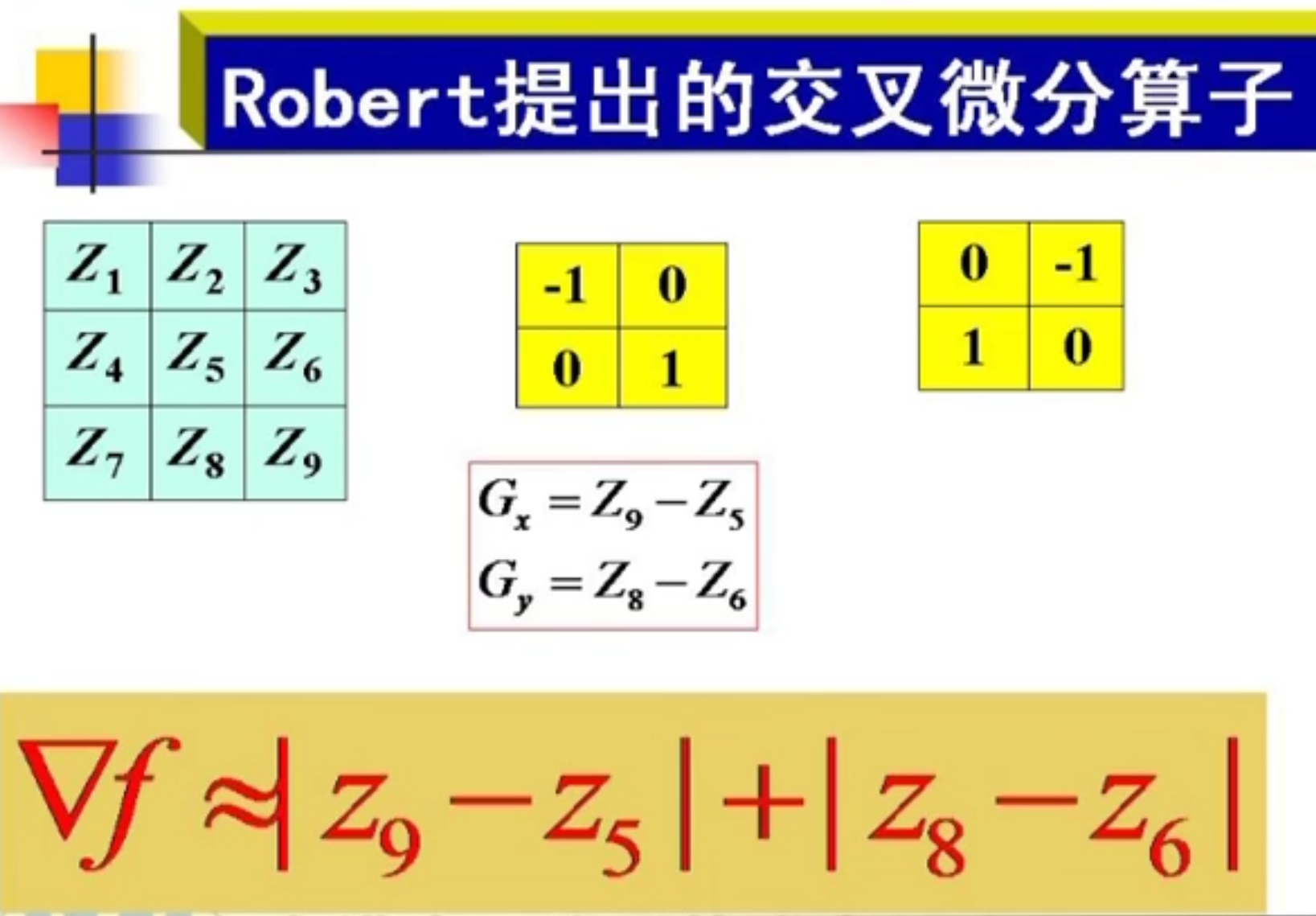### Sobel算子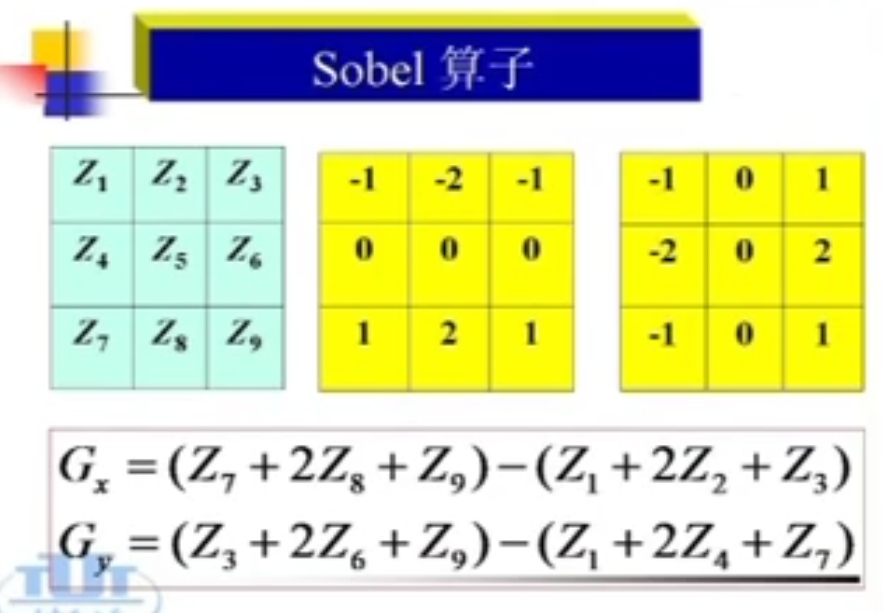Sobel算子既有平滑作用又有差分作用

### Prewitt算子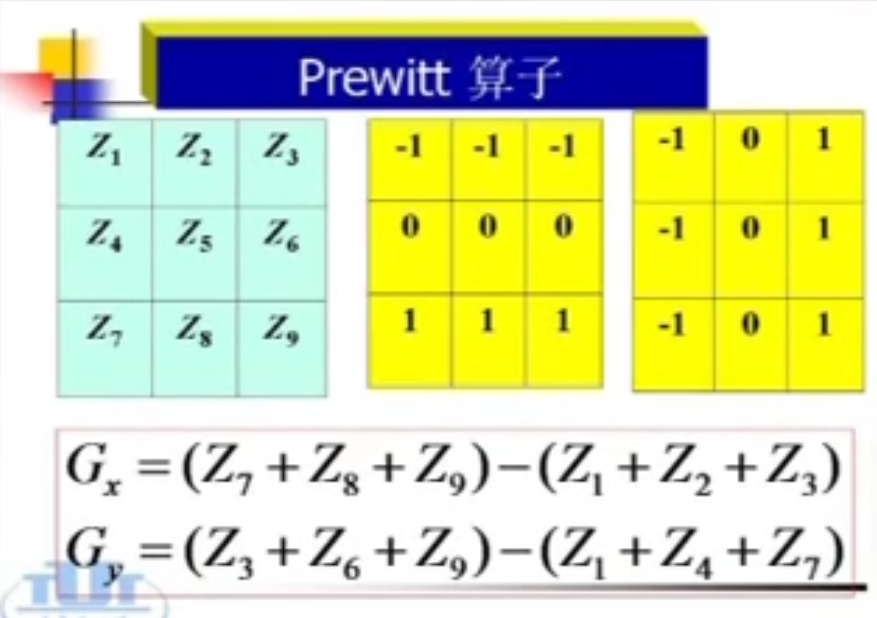sobel算子和Prewitt算子的区别在于系数，这些求梯度的运算可以利用卷积模板实现。

### 梯度锐化模板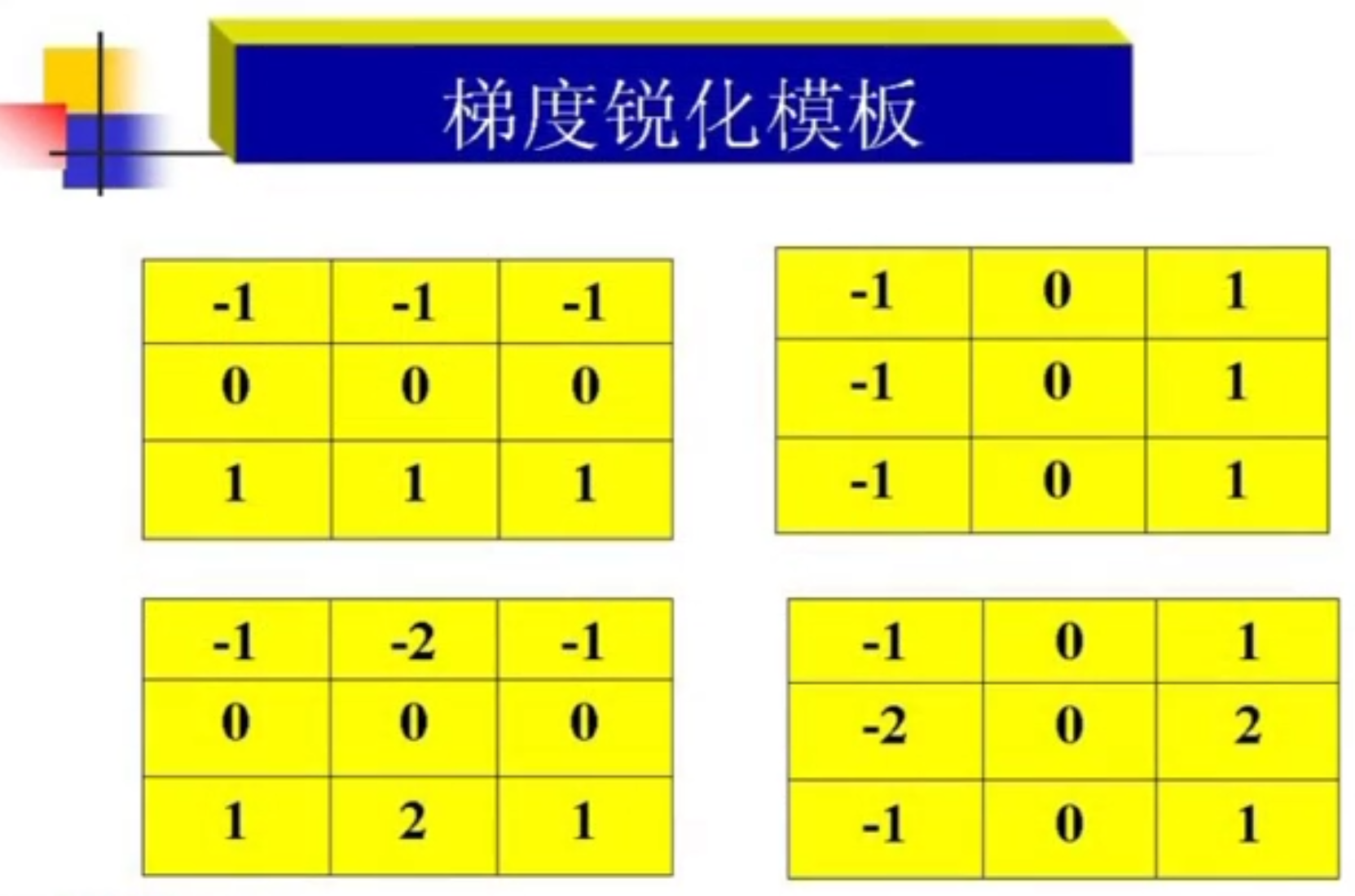## 二阶微分 --拉普拉斯算子

$\bigtriangledown^2 f = \frac{\partial^2 f}{\partial x^2}+\frac{\partial^2 f}{\partial y^2}$
x方向：
$\frac{\partial^2 f}{\partial x^2}=f(x+1)+f(x-1)-2f(x)$
y方向：
$\frac{\partial^2 f}{\partial y^2}=f(y+1)+f(y-1)-2f(y)$

$\bigtriangledown^2 f=[f(x+1,y)+f(x-1.y)+f(x,y+1)+f(x,y-1)]-4f(x,y)$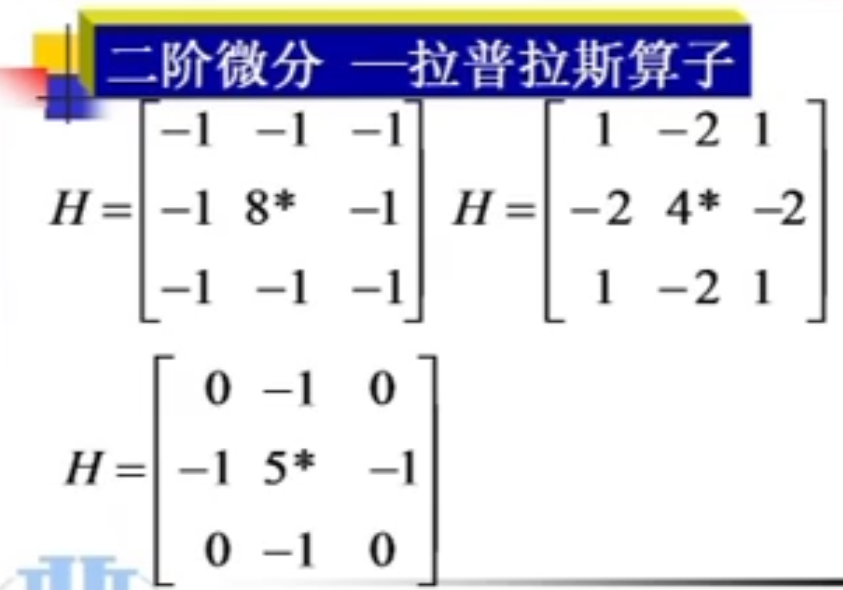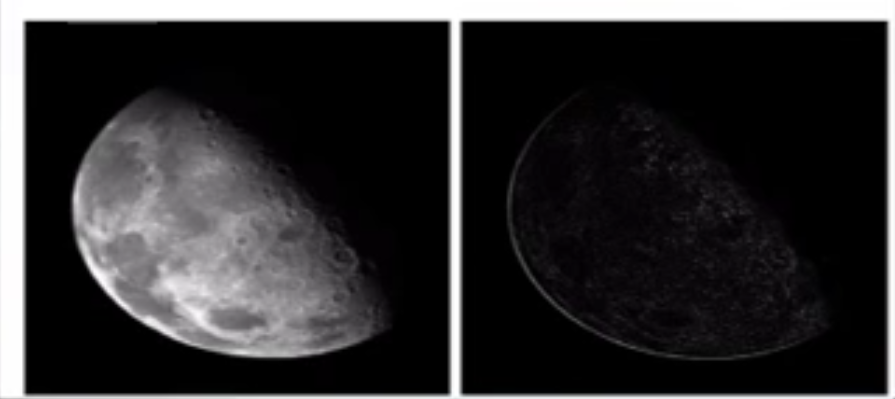### 高斯-拉普拉斯（log）算子

$\begin{bmatrix} -2 & -4 & -4 & -4 & -2 \\ -4 & 0& 8& 0& -4 \\ -4 & 8& 24^*& 8& -4 \\ -4 & 0& 8& 0& -4 \\ -2 & -4 & -4 & -4 & -2 \\ \end{bmatrix}$

## 高频提升滤波器

$g(x,y)=\alpha f(x,y) \pm \bigtriangledown f(x,y)$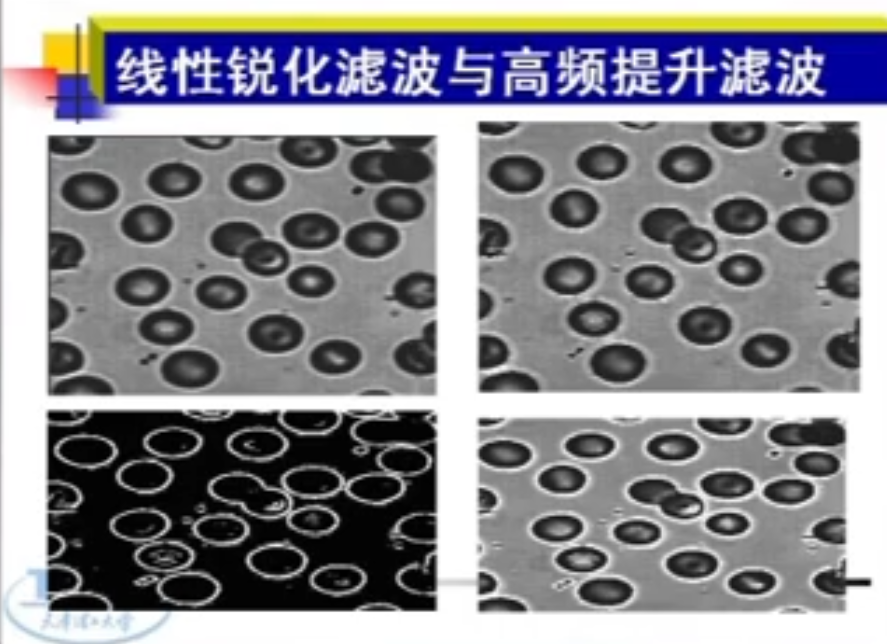## 自适应检测

### Kirsch边缘检测算子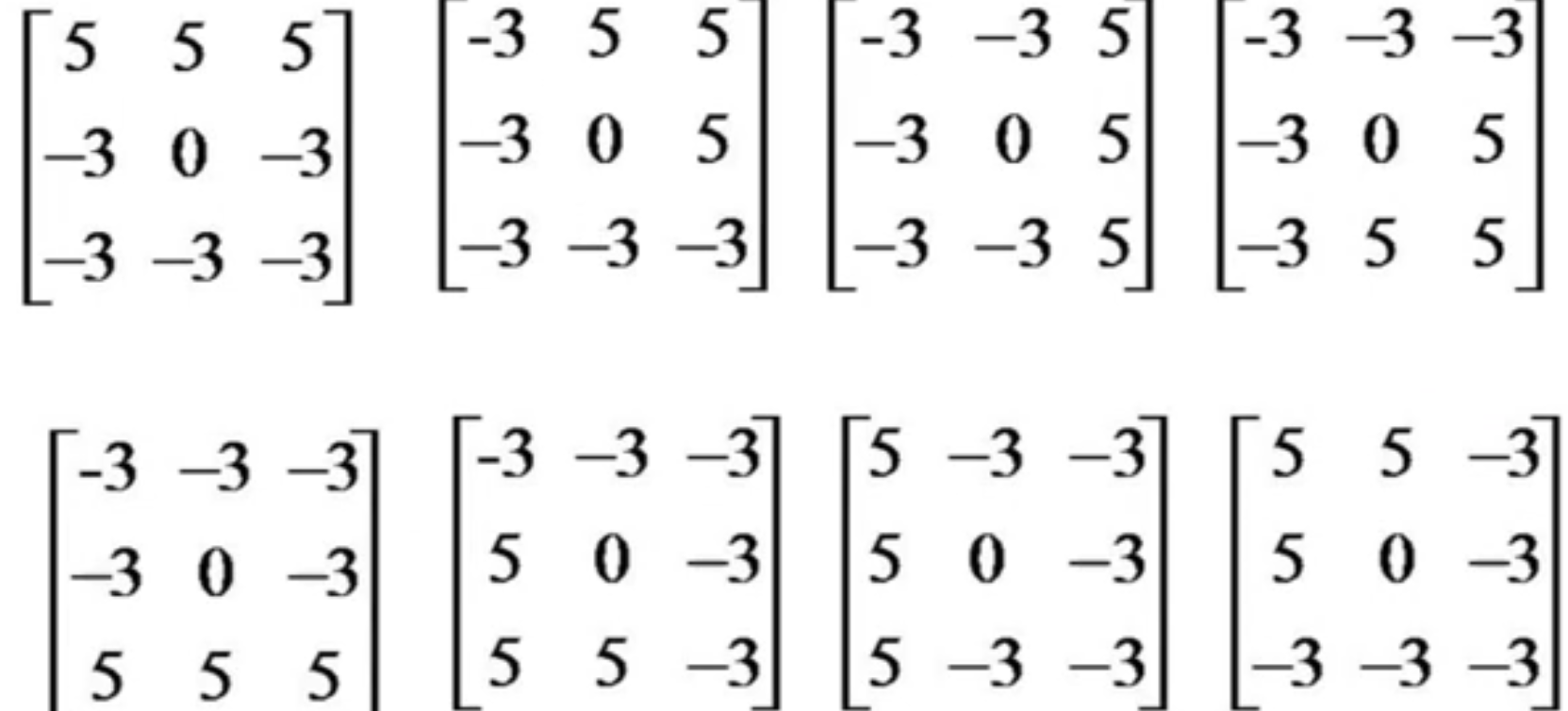Kirsch边缘检测算子检测的结果：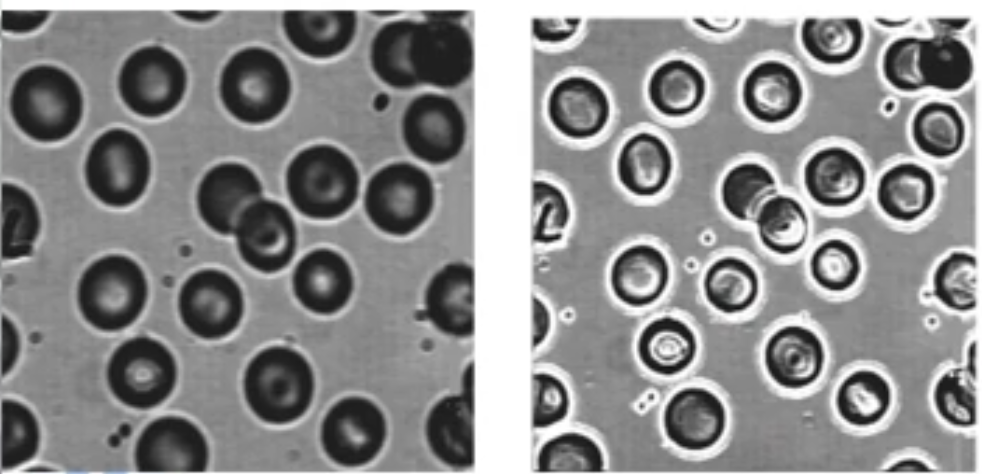## 锐化算子小结

• 好的检测结果：对边缘检测的错误率要低，在检测真实边缘的同时 ，避免检测出虚假的边缘。
• 好的边缘定位精度：标记的边缘位置要和图像上真正的边缘位置尽量接近；
• 对同一边缘要有低的响应次数：有的算子会对一个边缘产生多个相应，本来只有一共边缘点，可是检测出来会出现多个边缘点。要克服噪声的影响。

### Roberts算子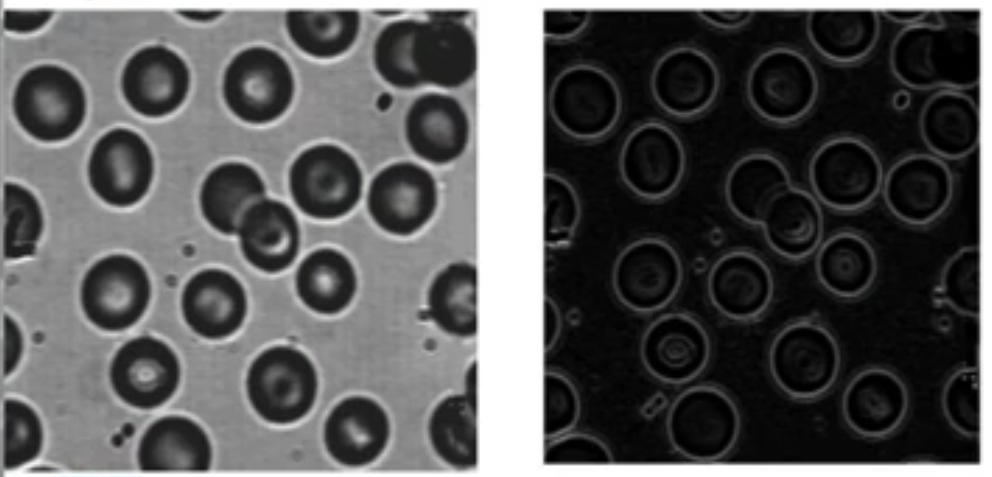### Sobel算子和Prewitt算子